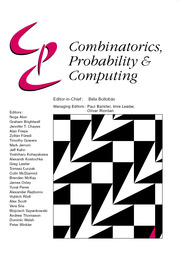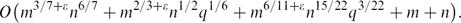Skip to main content Accessibility help
Home
Hostname: page-component-747cfc64b6-dkhcg Total loading time: 0.202 Render date: 2021-06-13T00:46:50.844Z Has data issue: true Feature Flags: { "shouldUseShareProductTool": true, "shouldUseHypothesis": true, "isUnsiloEnabled": true, "metricsAbstractViews": false, "figures": true, "newCiteModal": false, "newCitedByModal": true, "newEcommerce": true }Combinatorics, Probability and Computing

# Improved Bounds for Incidences Between Points and Circles

Published online by Cambridge University Press:  02 October 2014

Corresponding
E-mail address:

## Abstract

We establish an improved upper bound for the number of incidences between m points and n circles in three dimensions. The previous best known bound, originally established for the planar case and later extended to any dimension ≥ 2, is O*(m2/3n2/3 + m6/11n9/11 + m + n), where the O*(⋅) notation hides polylogarithmic factors. Since all the points and circles may lie on a common plane (or sphere), it is impossible to improve the bound in ℝ3 without first improving it in the plane.

Nevertheless, we show that if the set of circles is required to be ‘truly three-dimensional’ in the sense that no sphere or plane contains more than q of the circles, for some qn, then for any ϵ > 0 the bound can be improved to$O\bigl(m^{3/7+\eps}n^{6/7} + m^{2/3+\eps}n^{1/2}q^{1/6} + m^{6/11+\eps}n^{15/22}q^{3/22} + m + n\bigr).$
For various ranges of parameters (e.g., when m = Θ(n) and q = o(n7/9)), this bound is smaller than the lower bound Ω*(m2/3n2/3 + m + n), which holds in two dimensions.

We present several extensions and applications of the new bound.

1. (i) For the special case where all the circles have the same radius, we obtain the improved bound O(m5/11+ϵn9/11 + m2/3+ϵn1/2q1/6 + m + n).

2. (ii) We present an improved analysis that removes the subpolynomial factors from the bound when m = O(n3/2−ϵ) for any fixed ϵ < 0.

3. (iii) We use our results to obtain the improved bound O(m15/7) for the number of mutually similar triangles determined by any set of m points in ℝ3.

Our result is obtained by applying the polynomial partitioning technique of Guth and Katz using a constant-degree partitioning polynomial (as was also recently used by Solymosi and Tao). We also rely on various additional tools from analytic, algebraic, and combinatorial geometry.

## Keywords

Type
Paper
Information
Combinatorics, Probability and Computing , May 2015 , pp. 490 - 520
Copyright
Copyright © Cambridge University Press 2014

## Access options

Get access to the full version of this content by using one of the access options below.

## References

Agarwal, P. K., Apfelbaum, R., Purdy, G. and Sharir, M. (2007) Similar simplices in a d-dimensional point set. In Proc. 23rd ACM Symposium on Computational Geometry, pp. 232–238.Google Scholar
Agarwal, P. K., Matoušek, J. and Sharir, M. (2013) On range searching with semialgebraic sets~II. SIAM J. Comput. 42 20392062.CrossRefGoogle Scholar
Agarwal, P., Nevo, E., Pach, J., Pinchasi, R., Sharir, M. and Smorodinsky, S. (2004) Lenses in arrangements of pseudocircles and their applications. J. Assoc. Comput. Mach. 51 139186.CrossRefGoogle Scholar
Akutsu, T., Tamaki, H. and Tokuyama, T. (1998) Distribution of distances and triangles in a point set and algorithms for computing the largest common point sets. Discrete Comput. Geom. 20 307331.CrossRefGoogle Scholar
Alon, N. (1995) Tools from higher algebra. In Handbook of Combinatorics, Vols~1, 2, Elsevier, pp. 17491783.Google Scholar
Apfelbaum, R. and Sharir, M. (2011) Non-degenerate spheres in three dimensions. Combin. Probab. Comput. 20 503512.CrossRefGoogle Scholar
Aronov, B., Koltun, V. and Sharir, M. (2005) Incidences between points and circles in three and higher dimensions, Discrete Comput. Geom. 33 185206.CrossRefGoogle Scholar
Aronov, B. and Sharir, M. (2002) Cutting circles into pseudo-segments and improved bounds for incidences. Discrete Comput. Geom. 28 475490.CrossRefGoogle Scholar
Basu, S. and Sombra, M. Polynomial partitioning on varieties and point–hypersurface incidences in four dimensions. arXiv:1406.2144.Google Scholar
Beauville, A. (1996) Complex Algebraic Surfaces, second edition, Vol. 34 of London Mathematical Society Student Texts, Cambridge University Press.CrossRefGoogle Scholar
Bochnak, J., Coste, M. and Roy, M. (1998) Real Algebraic Geometry, Springer.CrossRefGoogle Scholar
Brass, P. (2002) Combinatorial geometry problems in pattern recognition. Discrete Comput. Geom. 28 495510.CrossRefGoogle Scholar
Chazelle, B., Edelsbrunner, H., Guibas, L. J., Sharir, M. and Stolfi, J. (1996) Lines in space: Combinatorics and algorithms. Algorithmica 15 428447.CrossRefGoogle Scholar
Cox, D., Little, J. and O'Shea, D. (2004) Using Algebraic Geometry, second edition, Springer.Google Scholar
Cox, D., Little, J. and O'Shea, D. (2007) Ideals, Varieties, and Algorithms: An Introduction to Computational Algebraic Geometry and Commutative Algebra, third edition, Springer.CrossRefGoogle Scholar
Elekes, G., Kaplan, H. and Sharir, M. (2011) On lines, joints, and incidences in three dimensions. J. Combin. Theory Ser. A 118 962977.CrossRefGoogle Scholar
Elekes, G. and Sharir, M. (2011) Incidences in three dimensions and distinct distances in the plane. Combin. Probab. Comput. 20 571608.CrossRefGoogle Scholar
Erdős, P. (1946) On sets of distances of n points. Amer. Math. Monthly 53 248250.CrossRefGoogle Scholar
Fox, J., Pach, J., Sheffer, A., Suk, A. and Zahl, J. A semi-algebraic version of Zarankiewicz's problem, arXiv:1407.5705.Google Scholar
Fuchs, D. and Tabachnikov, S. (2007) Mathematical Omnibus: Thirty Lectures on Classical Mathematics, AMS.CrossRefGoogle Scholar
Fulton, W. (1998) Intersection Theory, Springer.CrossRefGoogle Scholar
Guth, L. Distinct distance estimates and low degree polynomial partitioning. arXiv:1404.2321.Google Scholar
Guth, L. and Katz, N. H. (2010) Algebraic methods in discrete analogs of the Kakeya problem. Adv. Math. 225 28282839.CrossRefGoogle Scholar
Guth, L. and Katz, N. H. On the Erdős distinct distances problem in the plane. Ann. of Math., to appear. arXiv:1011.4105.Google Scholar
Harris, J. (1992) Algebraic Geometry: A First Course, Springer.CrossRefGoogle Scholar
Hartshorne, R. (1983) Algebraic Geometry, Springer.Google Scholar
Hartshorne, R. (2000) Geometry: Euclid and Beyond, Springer.CrossRefGoogle Scholar
Hwang, J. M. (2005) A bound on the number of curves of a given degree through a general point of a projective variety. Compositio Math. 141 703712.CrossRefGoogle Scholar
Kaplan, H., Matoušek, J., Safernová, Z. and Sharir, M. (2012) Unit distances in three dimensions. Combin. Probab. Comput. 21 597610.CrossRefGoogle Scholar
Kaplan, H., Matoušek, J. and Sharir, M. (2012) Simple proofs of classical theorems in discrete geometry via the Guth–Katz polynomial partitioning technique. Discrete Comput. Geom. 48 499517.CrossRefGoogle Scholar
Kaplan, H., Sharir, M. and Shustin, E. (2010) On lines and joints. Discrete Comput. Geom. 44 838843.CrossRefGoogle Scholar
Landsberg, J. M. (1999) Is a linear space contained in a submanifold? On the number of derivatives needed to tell. J. Reine Angew. Math. 508 5360.CrossRefGoogle Scholar
Landsberg, J. M. (2003) Lines on projective varieties. J. Reine Angew. Math. 562 13.CrossRefGoogle Scholar
Marcus, A. and Tardos, G. (2006) Intersection reverse sequences and geometric applications. J. Combin. Theory Ser. A 113 675691.CrossRefGoogle Scholar
Matoušek, J. (2002) Lectures on Discrete Geometry, Springer.CrossRefGoogle Scholar
Milnor, J. (1964) On the Betti numbers of real varieties. Proc. Amer. Math. Soc. 15 275280.CrossRefGoogle Scholar
Miranda, R. (1995) Algebraic Curves and Riemann Surfaces, Vol. 5 of Graduate Studies in Mathematics, AMS.CrossRefGoogle Scholar
Nilov, F. and Skopenkov, M. (2013) A surface containing a line and a circle through each point is a quadric. Geom. Dedicata 163 301310.CrossRefGoogle Scholar
Pach, J. and Sharir, M. (2004) Geometric incidences. In Towards a Theory of Geometric Graphs (Pach, J., ed.), Vol. 342 of Contemporary Mathematics, AMS, pp. 185223.CrossRefGoogle Scholar
Quilodrán, R. (2010) The joints problem in Rn. SIAM J. Discrete Math. 23 22112213.CrossRefGoogle Scholar
Roy, M.-F. and Vorobjov, N. (2002) The complexification and degree of a semi-algebraic set. Math. Z. 239 131142.CrossRefGoogle Scholar
Salmon, G. (1915) A Treatise on the Analytic Geometry of Three Dimensions, Vol. 2, fifth edition, Hodges, Figgis and Co. Ltd., Dublin.Google Scholar
Sard, A. (1942) The measure of the critical values of differentiable maps. Bull. Amer. Math. Soc. 48 883890.CrossRefGoogle Scholar
Sharir, M. and Solomon, N. (2014) Incidences between points and lines in four dimensions. In Proc. 30th ACM Symposium on Computational Geometry, 189–197.Google Scholar
Solymosi, J. and Tao, T. (2012) An incidence theorem in higher dimensions. Discrete Comput. Geom. 48 255280.CrossRefGoogle Scholar
Székely, L. (1997) Crossing numbers and hard Erdős problems in discrete geometry. Combin. Probab. Comput. 6 353358.CrossRefGoogle Scholar
Thom, R. (1965) Sur l'homologie des variétés algebriques réelles. In Differential and Combinatorial Topology (Cairns, S. S., ed.), Princeton University Press, pp. 255265.Google Scholar
Warren, H. E. (1968) Lower bound for approximation by nonlinear manifolds. Trans. Amer. Math. Soc. 133 167178.CrossRefGoogle Scholar
Whitney, H. (1957) Elementary structure of real algebraic varieties. Ann. of Math. 66 545556.CrossRefGoogle Scholar
Zahl, J. (2013) An improved bound on the number of point–surface incidences in three dimensions. Contrib. Discrete Math. 8 100121.Google Scholar
Zahl, J. A Szemerédi–Trotter type theorem in ℝ4. arXiv:1203.4600.Google Scholar
10
Cited by

# Send article to Kindle

To send this article to your Kindle, first ensure no-reply@cambridge.org is added to your Approved Personal Document E-mail List under your Personal Document Settings on the Manage Your Content and Devices page of your Amazon account. Then enter the ‘name’ part of your Kindle email address below. Find out more about sending to your Kindle. Find out more about sending to your Kindle.

Note you can select to send to either the @free.kindle.com or @kindle.com variations. ‘@free.kindle.com’ emails are free but can only be sent to your device when it is connected to wi-fi. ‘@kindle.com’ emails can be delivered even when you are not connected to wi-fi, but note that service fees apply.

Find out more about the Kindle Personal Document Service.

Improved Bounds for Incidences Between Points and Circles
Available formats
×

# Send article to Dropbox

To send this article to your Dropbox account, please select one or more formats and confirm that you agree to abide by our usage policies. If this is the first time you use this feature, you will be asked to authorise Cambridge Core to connect with your <service> account. Find out more about sending content to Dropbox.

Improved Bounds for Incidences Between Points and Circles
Available formats
×

# Send article to Google Drive

To send this article to your Google Drive account, please select one or more formats and confirm that you agree to abide by our usage policies. If this is the first time you use this feature, you will be asked to authorise Cambridge Core to connect with your <service> account. Find out more about sending content to Google Drive.

Improved Bounds for Incidences Between Points and Circles
Available formats
×
×

#### Reply to:Submit a response

Please enter your response.

#### Your details

Please enter a valid email address.

#### Conflicting interests

Do you have any conflicting interests? *